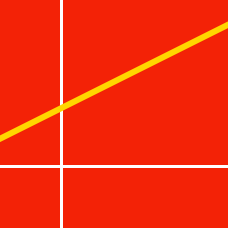Geometry

# Equation of a Line: Level 4 Challenges

Given that a straight line in the $xy$-plane passes through the point $(3,48)$ in the first quadrant and intersects the positive $x$- and $y$-axes at points $P$ and $Q$ respectively. Let $O$ designate the origin.

Find the minimum value of $OP\times OQ$.

The line $4x + y = 1$ passes through the point $A(2,-7)$ and intersects point $B$ on the line $BC$ whose equation is $3x - 4y + 1=0$. The equation of the line $AC$ is $ax + by + c = 0$ (with $a,$ $b,$ and $c$ all coprime) and constructed so that the distance from $A$ to $B$ is the same as the distance from $A$ to $C$.

What is the minimum positive value of $a+b+c$?

Note: $A,B,C$ are all different points in the coordinate plane.

$6x^2-\alpha xy-3y^2-24x+3y+\beta=0$

The equation above represents a pair of lines that intersect on the $x$-axis. Find the value of $20\alpha-\beta$.

There exist two ordered triples $(a,b,c)$ for which $4x^2-4xy+ay^2+bx+cy+1=0$ represents a pair of identical straight lines in the $xy$-plane.

If these triples are $(a_1,b_1,c_1)$ and $(a_2,b_2,c_2)$, then find the value of $a_1+b_1+c_1+a_2+b_2+c_2$.

Given that a straight line in the $xy$-plane passes through the point $(3,48)$ in the first quadrant and intersects positive $x$- and $y$-axes at points $P$ and $Q$ respectively. Let $O$ designate the origin.

Find the minimum value of $OP+OQ$.

×